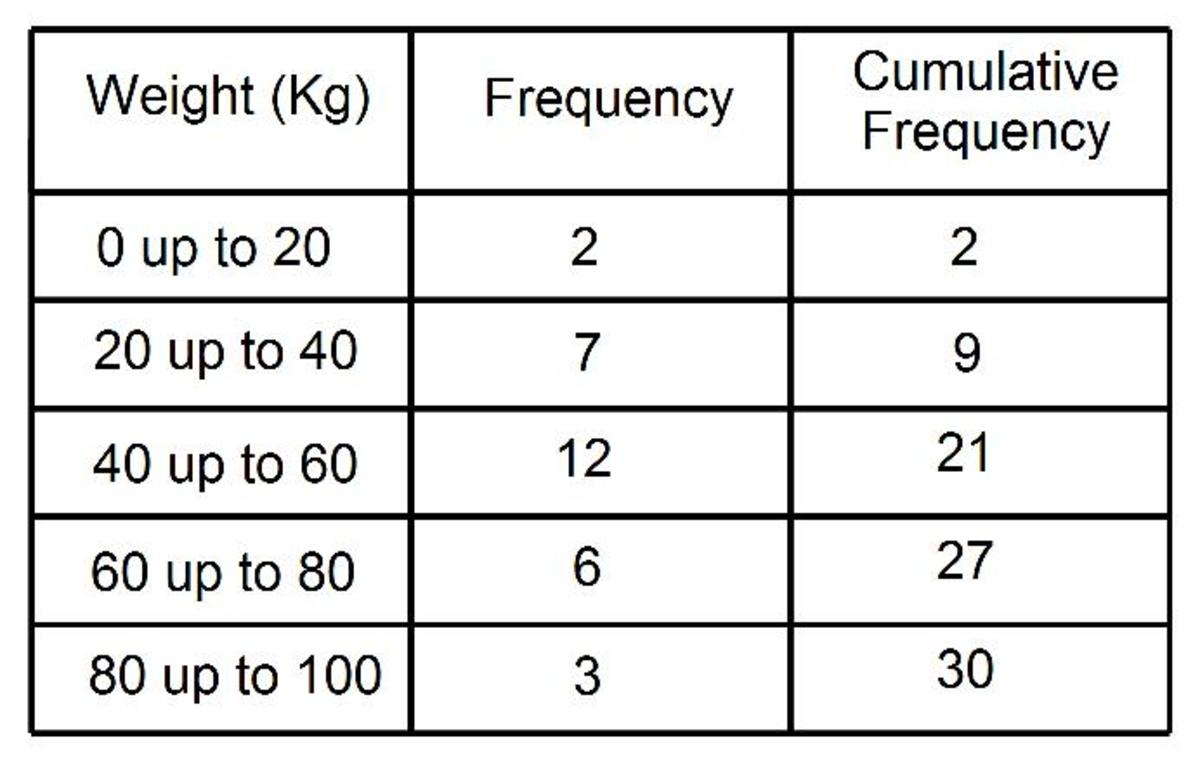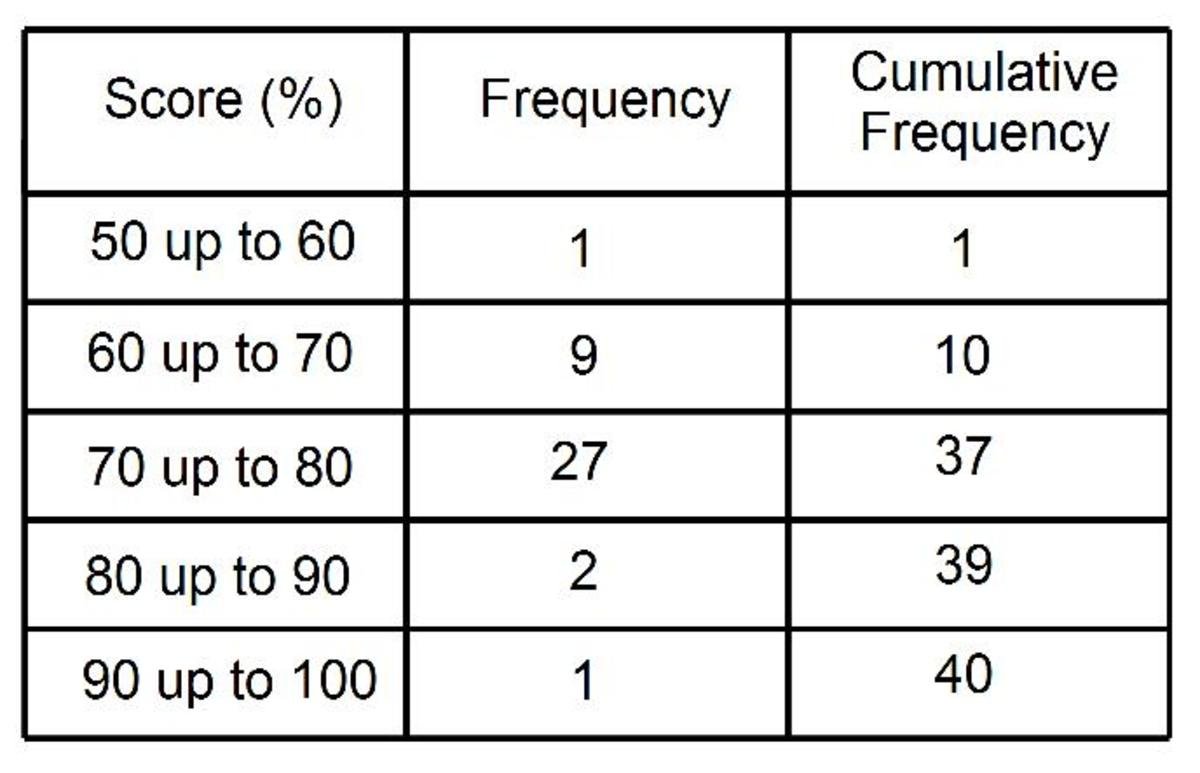Updated date:

# Cumulative Frequency Tables. How to work out the the cumulative frequencies from a frequency table.

Author:The cumulative frequency can be calculated by adding up the frequencies in the frequency table. Start by writing the first frequency cumulative frequency columns and keep adding on the next frequency onto the last cumulative frequency.

Lets’ take a look example:

The frequency table shows the weights of a group of people. 2 people have a weight between 0 and 20kg, 7 people have a weight between 20 and 40kg, 12 people have a weight between 40 and 60kg, 6 people have a weight between 60 and 80kg and 3 people have a weight between 80 and 100kg. Work out the cumulative frequencies:

The first number in the cumulative frequency column is 2 because 2 people have a weight up to 20kg.

The next number in the cumulative frequency column is 9 because 9 people have a weight up to 40kg (2 + 7).

The next number in the cumulative frequency column is 21 because 21 people have a weight up to 60kg (2 + 7 + 12).

The next number in the cumulative frequency column is 27 because 27 people have a weight up to 80kg (2 + 7 + 12 + 6).

The final value in the cumulative frequency columns is 30 because 30 people have a weight up to 100 kg (2 + 7 + 12 + 6 + 3).Let’s look at another example of working out the cumulative frequencies.

This next frequency table shows the test results of a group of students. Work out the cumulative frequencies.

1 person scored between 50% and 60%, 9 people scored between 60% and 70%, 27 people scored between 70% and 80%, 2 people scored between 80% and 90% and 1 person scored between 90% and 100%. Work out the cumulative frequencies:

The first number in the cumulative frequency column is 1 because 1 person scored up to 60%.

The next number in the cumulative frequency column is 10 because 10 people scored up to 70% (1 + 9).

The next number in the cumulative frequency column is 37 because 37 people scored up to 80% (1 + 9 + 27).

The next number in the cumulative frequency column is 39 because 39 people scored up to 90% (1 + 9 + 27 + 2).

The final value in the cumulative frequency columns is 40 because 40 people scored up to 100% (1 + 9 + 27 + 2 + 1).

Once the cumulative frequency values have been work out you can go onto draw the cumulative frequency graph.

To see an example on drawing a cumulative frequency graph check out the article that follows.

Cumulative Frequency Graphs (Polygon or Curve) And How To Find the LQ, Median, UQ and IQR.